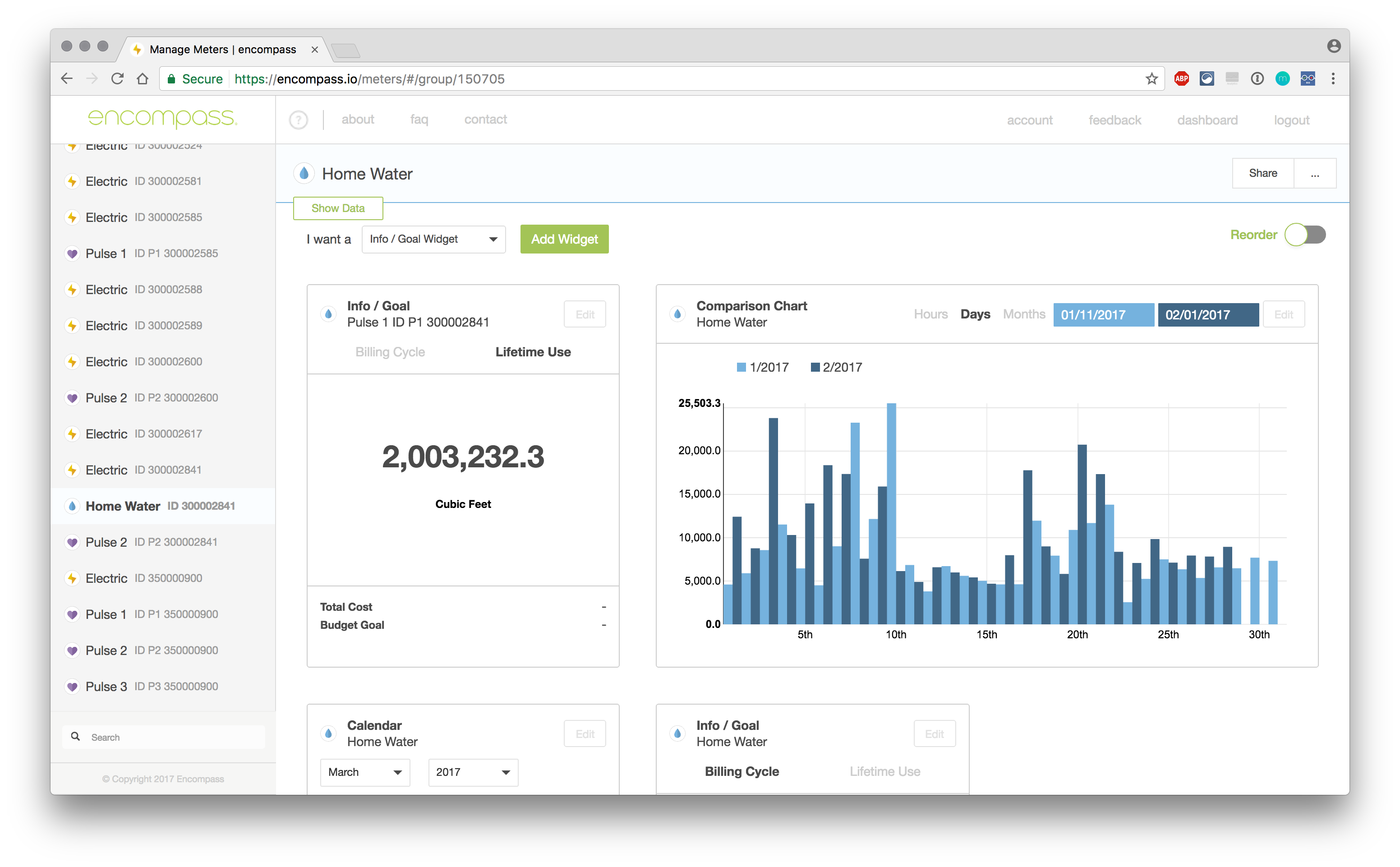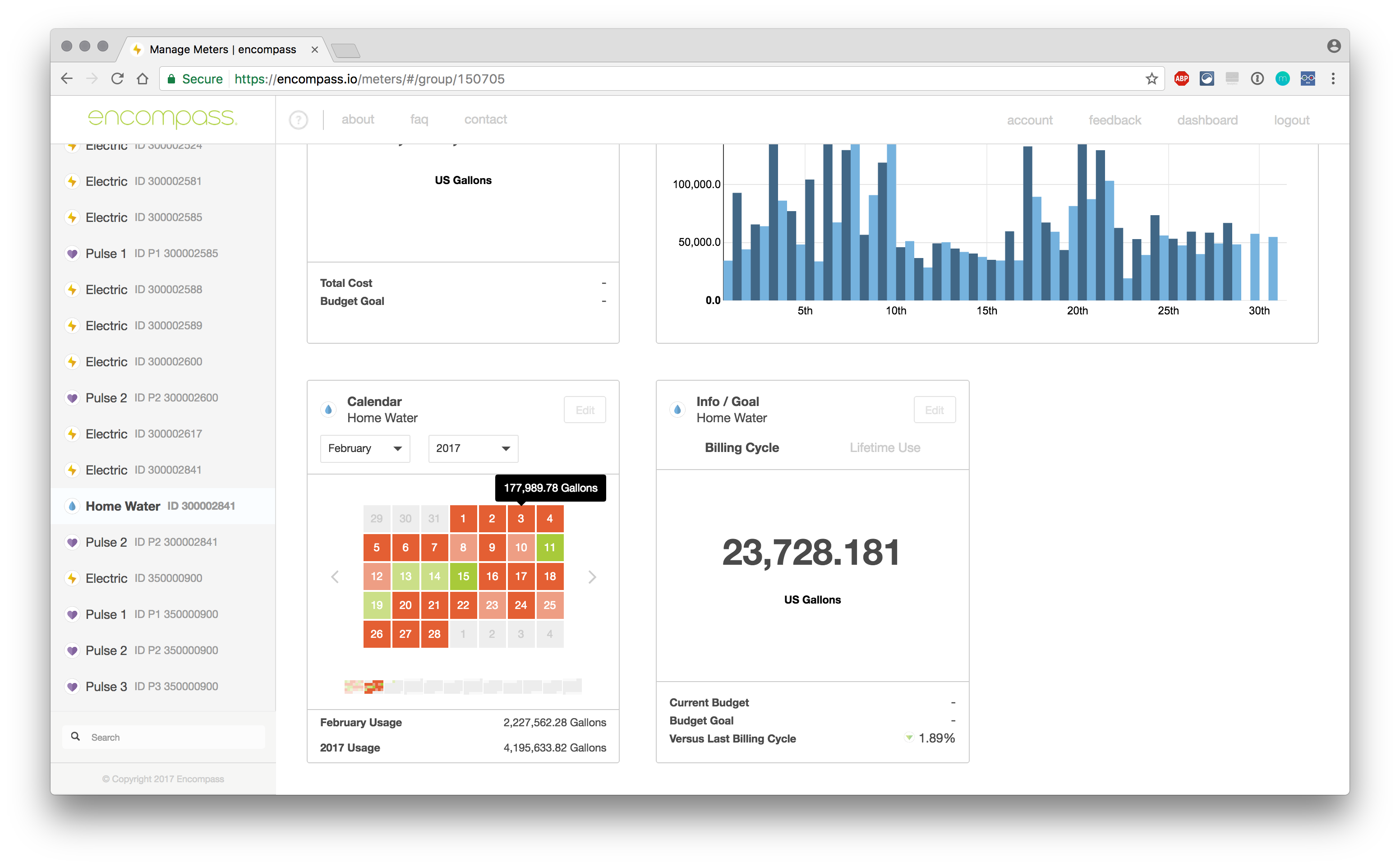All of our Water Meters read natively in cubic feet.

Converting to another unit of measurement is a simple matter of multiplication. To convert from Cubic Feet to Gallons you multiply your meter's reading (A) by 7.48052

Here is a list of equations and multipliers to convert from any amount of cubic feet (A) to an amount in another unit of measurement (B):

(A) x 7.48052 = (B) US Gallons

(A) x 1728 = (B) Cubic Inches

(A) x 0.0283168 = (B) Cubic Meters

(A) x 28.3168 = (B) Liters

If you are reading our water meters remotely with an Omnimeter Pulse v.4 and EKM Push3, you can use Encompass.io to convert from the cubic feet that our water meters natively read in, to various other units including gallons.